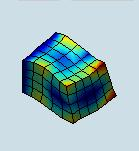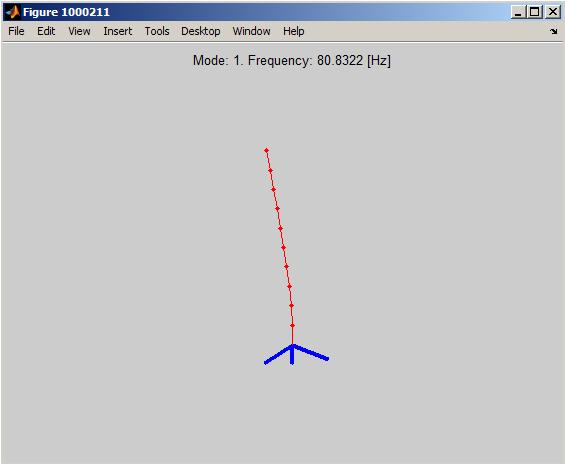Date:
 3D beam modal analysis using D3_BEAM element .    3D beam input file    ``` Dimensions: length = 1.0 m, width = 0.1 m, height = 0.1 m. Material properties: E = 2e11 Pa, ρ = 7890 kg/m3, ν = 0.3. ``` ``` Theoretical frequency for the first mode: 81 Hz ``` ``` Computed 1st modal frequency: 80.83 Hz```